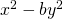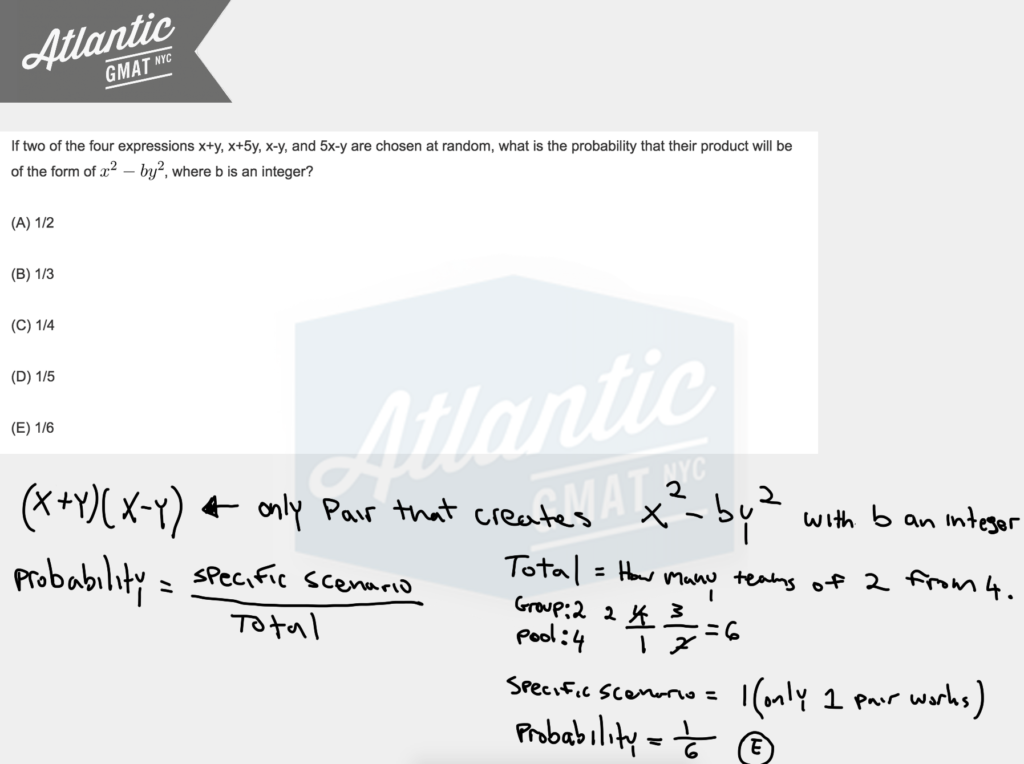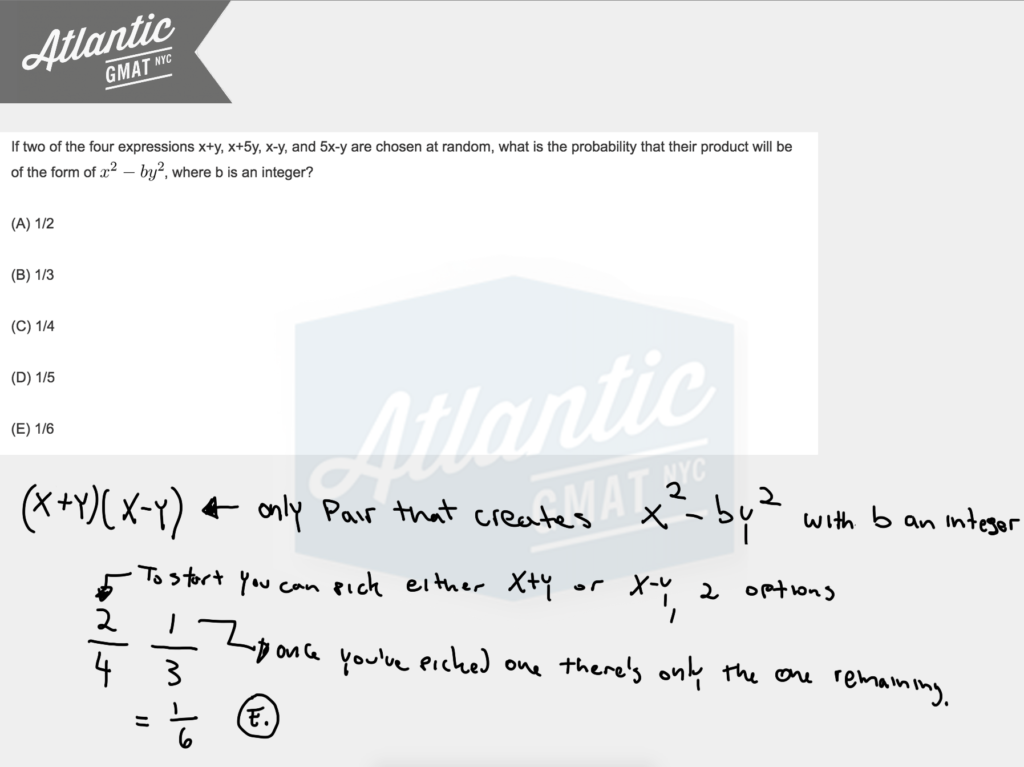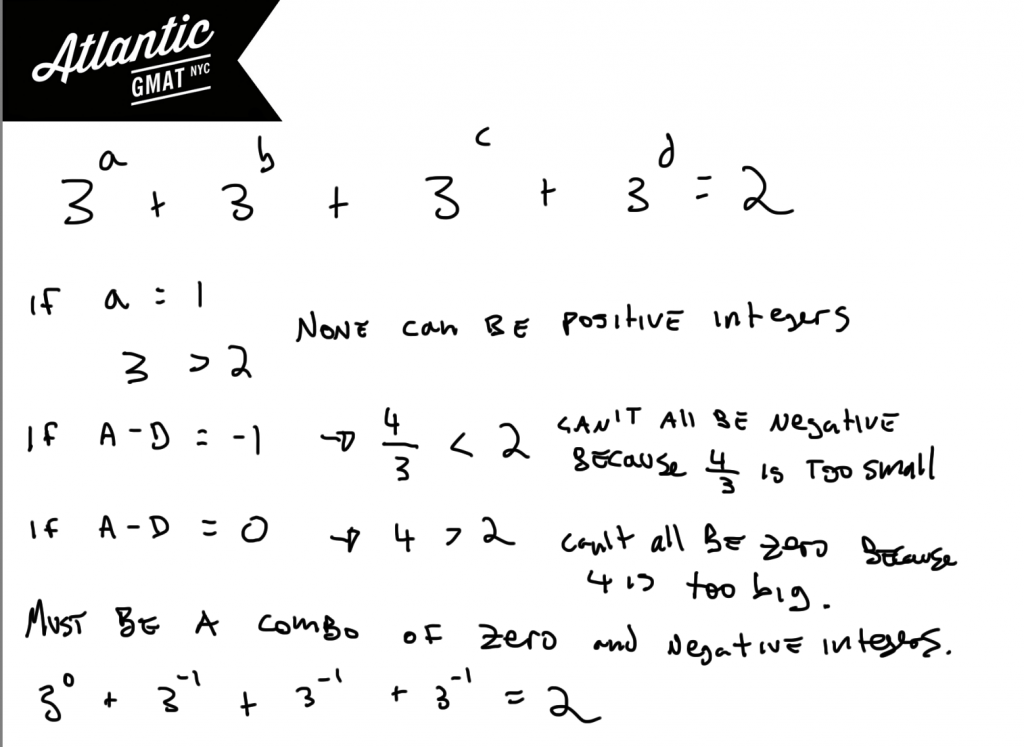Private GMAT Tutoring in NYC & Online

If M = √4 + 4^1/3 + 4^1/4, then the value of M is:

(A) Less than 3
(B) Equal to 3
(C) Between 3 and 4
(D) Equal to 4
(E) Greater than 4

When a certain tree was first planted, it was 4 feet tall and the height of the tree increased by a constant amount each year for the next 6 years. At the end of 6 year the tree was 1/5 taller than it was at the end of 4 year. By how many feet did the height of the tree increase each year

(A) 3/10
(B) 2/5
(C) 1/2
(D) 2/3
(E) 6/5

The "tree" questions comes up about 50% of the time in GMAT tutoring sessions. There's nothing crazy going on here but you do need to focus on the question and then stay organized in the follow through.

I'd go ahead and draw a little chart to make sense of the information. When the tree was first planted it was 4 feet tall so that's the start of the number line and then it grew by a constant amount each year so assign a variable for that, say x. Then you can define the 6th year in terms of the 4th year (the 6th year is 5/4ths the 4th year). That's it. Solve for x.

If n denotes a number to the left of 0 on the number line such that the square of n is less than 1/100, then the reciprocal of n must be

(A) Less than -10
(B) Between -1 and -1/10
(C) Between -1/10 and 0
(D) Between 0 and 1/10
(E) Greater than 10

If two of the four expressions x+y, x+5y, x-y, and 5x-y are chosen at random, what is the probability that their product will be of the form of, where b is an integer?

(A) 1/2

(B) 1/3

(C) 1/4

(D) 1/5

(E) 1/6

Be super comfortable with difference of squares. You're almost guaranteed to need it on your GMAT. You need to know it forwards, backwards, and upside-down. There isn't much content you need to know for the GMAT quant but in order to be consistently successful so that you can go in there on test day and snag your 700+ score you need complete fluency with that limited content. On this one we're looking for a product of two terms that will produce difference of squares. So we need something in the format: (x+y(x-y). There's only one pairing that gives us the right format. Now you have to figure out what that means in terms of probability. In general, probability is specific scenario/total scenarios. You can calculate the total and the specific scenarios using the slot method. How many teams of 2 can you make from four things? (4*3)/(1*2) = 6. So that's your denominator. And then there's only one team that works, the pairing of (x + y)(x - y). So that leaves you with 1/6. E. Detailed diagrams below and an in depth video explantation here: If two of the four expressionsYou also can think about this in terms of straight probability and say: 2 choices our of 4 and then 1 choice out of 3. 2/4 * 1/3 = 2/12 = 1/6. Comment with any questions or additions. Happy studies!# GMAT Question of the Day - Problem Solving - Exponents



If a, b, c, and d are integers then what is the value of a + b + c + d?

A. -3

B. -2

C. -1

D. 1

E. 2

# GMAT Question of the Day Solution

If you try algebra on this GMAT question of the day you'll have some issues. This isn't solvable in a conventional way. This is a "perfect fit" type of question. What's that??? The GMAT will set up a scenario which isn't solvable algebraically but for which there is a unique solution. Whoa!!??? Yep. So how do you get there? ORGANIZATION and a little imagination. Consider the limitations of the system presented to you. Are there any special numbers? Do the values have to be integers? What are the implications of that? Rest assured that the question is generally simpler than you think it is.

In this case we do have integer values for a, b, c, and d. That limits the possibilities by a ton. Now notice that these four powers of three must equal 2. Hmmm. That should make you scratch your head. How is that possible? Well, consider the values that you can use. What happens if you use positive integers? Negative integers? Zero (yes, zero is also an integer. Don't forget it!)? Positive integers won't work because even if one of the three's is raised to the 1st power you'll be above 2 (none of the other numbers can possibly be negative because none of the bases are negative). Making all the variables zero will put you squarely at 3. Still too big. Using the biggest negative integer (-1) will get you to 4/3. Too small and a fraction. This guarantees that we'll need a combination of zero and negative integers. If three of the variables equal -1 and one of them equals zero the result will sum to 2.

Another way to look at this is to think: if the sum of 4 numbers (none of which can be zero or negative) is 2 then at least 3 of the numbers must be less than 1. Because you need to end up with an integer the fractions must sum to an integer. The only one that will do that in this case is 1/3.# CONTACT

Atlantic GMAT

405 East 51st St.

NY, NY 10022

(347) 669-3545

info@AtlanticGMAT.com